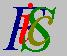Mauro Murzi's pages on Philosophy of Science - Logical Positivism
Observational and Theoretical Terms

# [2. The Main Philosophical Tenets of Logical Positivism.]

## d. Observational and Theoretical Terms.

The distinction between observational and theoretical terms depends on the Verifiability Principle. A statement is meaningful only if it is verifiable; however, in scientific theories, there are many statements which are not verifiable, for example, assertions dealing with quantum particles or relativistic gravitational fields. These statements are too abstract for a direct test; strictly speaking, they are meaningless. To avoid such a consequence, different approaches were proposed. According to Schlick, the principles of a scientific theory are not statements, but rules of inference, and so the problem of their meaning does not arise ("Die Kausalität in der gegenwärtigen Physik" in Die Naturwissenschaften, 19, 1931). Neurath proposed another solution: the terms which belong to the abstract language of a scientific theory are explicitly definable in a restricted language whose terms describe directly observable objects ("Physikalismus" in Scientia, 50, 1931). So a distinction between observational and theoretical terms became crucial. Carnap realized that theoretical terms are not definable by observational ones and he proposed a partial reducibility of theoretical to observational terms ("Testability and meaning" in Philosophy of Science, 3, 1936 and 4, 1937). However, it became evident that theoretical terms cannot be eliminated from scientific theories neither by means of definitions nor by means of rules of correspondence.

A result of formal logic, known as Craig's Interpolation Theorem, suggested a possible way in order to eliminate theoretical terms. Let A and B be two set of statements, so that B is a logical consequence of A. Craig proved that (i) there is a set C of statements whose terms are common to A and B, (ii) C is a logical consequence of A, and (iii) B is a logical consequence of C. If A were the set of axioms of a scientific theory and B were the set of observational statements implied by A, then there would be a set C, whose terms are the observational terms which occur in the axioms, and yet C would entail B. It follows that it is possible to translate a scientific theory in an observational language without any loss of deductive power. Given a theory T, it is thus possible to build a theory T* without theoretical terms so that T and T* are equivalent with respect to observational statements; that is, every observational statement O is a logical consequence of T if and only if it is a logical consequence of T*.

Hempel ("The Theoretician's Dilemma" in Minnesota Studies in the Philosophy of Science, II, 1958) noted that the theory T* without theoretical terms, in spite of the equivalence (with respect to the observational language) to the original theory T, is not as useful as T. In fact, from an inductive point of view, T and T* are very different. Usually the original theory T suggests certain relations between its concepts, while in T* these concepts are forbidden. The discovery of laws is almost impossible in T*, while it is a natural consequence in T. Moreover, while the number of the axioms of T usually is finite, Craig's Theorem does not assure us of the existence of a theory T* with a finite number of axioms. So T* is almost useless. Theoretical terms are thus necessary in science.

Hempel's "The Meaning of Theoretical Terms" in Logic, Methodology and Philosophy of Science IV, 1973, includes a new criticism of the observational-theoretical distinction. The two main points of Hempel's analysis are:

1. Observational terms do not exist. Only a distinction between terms used in a given theory and new terms employed for the first time in a new scientific theory is possible. For example, Bohr's atomic theory includes terms like quantum numbers, quantum jump, steady state, and explains spectra described with the help of wavelength. Now wavelength is an "old term" while quantum number is a "new term." Thus the abstract concepts of atomic theory are linked with other abstract (but already given) concepts.
2. The meaning of theoretical terms is not defined by analytic statements which are true by convention. In fact, every statement is subject to empirical tests. In a scientific theory, there is no room for "true by convention".

In Philosophical Foundations of Physics, 1966, Carnap proposed a slightly different approach to the observational-theoretical distinction. Now the starting-point is the difference between empirical and theoretical laws. It is possible to directly confirm (or disprove) an empirical law, while a theoretical law can be tested only through the empirical laws that are among its consequences. Moreover, an empirical law explains facts while a theoretical law explains empirical laws. Thus there are three levels:

1. Empirical facts.
2. Simple generalizations we can directly test, i.e. empirical laws. They explain facts and are employed to forecast facts.
3. General principles we can use to explain empirical laws, i.e. theoretical laws.

Empirical laws include observational terms, while theoretical terms occur in theoretical laws.